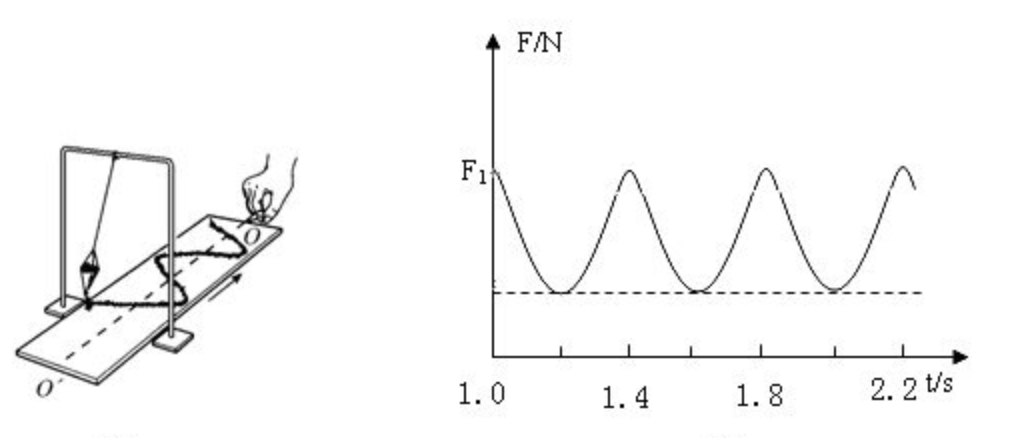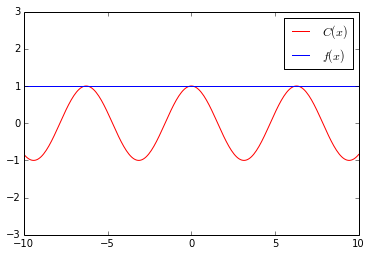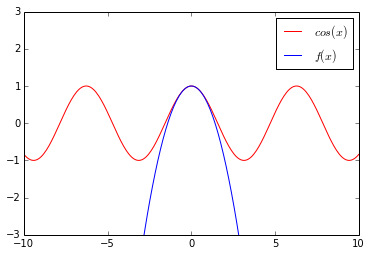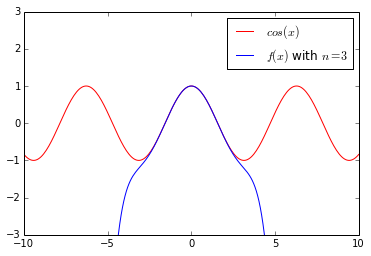# W.H.S.

## 1. 问题## 2. 局部模拟

$f(0) = a = C(0) = cos(0) = 1 \tag{3}$ $f^{'}(0) = b = C^{'}(0) = -sin(0) = 0 \tag{4}$$f(0) = a = C(0) = cos(0) = 1 \tag{7}$ $f^{'}(0) = b = C^{'}(0) = -sin(0) = 0 \tag{8}$ $f^{''}(0) = 2c = C^{''}(0) = -cos(0) = -1\tag{9}$## 3. 泰勒展开

\$f(x)(的k次导数为：## 【metric learning】Dense triplet loss

1. Input定义对于一个batch_siz为n， embedding特征长度为m的input，记为A:2. 计算距离矩阵对A进行elementwise square, 得到：然后对$$A^2$$按行做reduce sum, 得到：A乘上A的转置得：由等式（3）（4）得：等式（5）的计算结果即为我们要求的距离矩阵， 简单记为$$D$$：3. 计算Loss为了简单说明计算过程，我们假设Input的batch_size=6，而且有三个类别，其中： 类别0： $$x_0, x_1$$ 类别...…